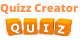Create Quiz

### Math

Quiz: Engineering Mathematics Mock Tests on Propositional and First Order Logic.
This quiz contains information about Engineering Mathematics Propositional and First Order Logic.
BYJack Smith8 months ago
Quiz: Engineering Mathematics Mock tests on Combinatorics.
This quiz contains Engineering Mathematics Combinatorics.
BYJack Smith8 months ago
Quiz: Engineering Mathematics Mock Tests on Graph Theory
This quiz contains information about Engineering Mathematics Graph Theory.
BYJack Smith8 months ago
Quiz: Engineering Mathematics Mock Tests on Numerical Methods and Calculus.
This quiz contains information about Engineering Mathematics Numerical Methods and Calculus.
BYJack Smith8 months ago
Quiz: Engineering Mathematics Mock tests on Linear Algebra.
This quiz contains information about Engineering Mathematics on Linear Algebra.
BYJack Smith8 months ago
Quiz: Engineering Mathematics Mock Tests on Set Theory and Algebra.
This quiz informs us about Engineering Mathematics et Theory & Algebra.
BYJack Smith9 months ago
ASVAB quiz
The two ASVAB tests pertaining to math are Arithmetic Reasoning and Math Knowledge. Arithmetic Reasoning is the ability to solve arithmetic word problems. Math Knowledge is the knowledge of high school mathematics principles.
BYJames Harbor9 months ago
GMAT math quiz
There are two main differences between GMAT math and high school math: GMAT math is easier! The hardest parts of high school math—calculus and trigonometry (sine, cosine, tangent, etc.)—are not even tested on the GMAT.
BYJames Harbor9 months ago